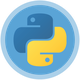Pypy Vs Python April 1, 2016, 5:05 p.m.

## Arm - Beagleboard

Calculation Table (in seconds) Python PyPy
Array Copy 89.420 19.510
Float Arithmetic 77.180 0.640
Integer Arithmetic 75.720 6.270
String Search 14.860 0.640
Total 257.210 27.070

## 80x86 64bit (4 core but single thread)

Calculation Table (in seconds) Python PyPy
Array Copy 26.906 8.053
Float Arithmetic 25.386 0.178
Integer Arithmetic 23.494 1.988
String Search 4.856 0.219
Total 80.642 10.438

## Test Code:

``````# -*- coding: utf-8 -*-
import time

__author__ = 'ozgur'
__creation_date__ = '4/1/16' '4:11 PM'

TEST_STRING = '''The hierarchy shown above is relative to a PREFIX directory. PREFIX is computed by starting from the directory where the executable resides, and “walking up” the filesystem until we find a directory containing lib_pypy and lib-python/2.7.
The archives (.tar.bz2 or .zip) containing PyPy releases already contain the correct hierarchy, so to run PyPy it’s enough to unpack the archive, and run the bin/pypy executable.
To install PyPy system wide on unix-like systems, it is recommended to put the whole hierarchy alone (e.g. in /opt/pypy2.1) and put a symlink to the pypy executable into /usr/bin or /usr/local/bin
If the executable fails to find suitable libraries, it will report debug: WARNING: library path not found, using compiled-in sys.path and then attempt to continue normally. If the default path is usable, most code will be fine. However, the sys.prefix will be unset and some existing libraries assume beni bul that this is never the case.'''

class StressSuite():
def __init__(self):
pass

@staticmethod
def test_array():
a = []
for y in range(1000000):
txt_arr = "deneme".split()
for item in txt_arr:
a.append(item)
del (a)

@staticmethod
def test_float():
a = 0.5612342
b = 653.324556
c = a + b
d = a * b
e = b / a
f = a - b

@staticmethod
def test_int():
a = 5612342
b = 653324556
c = a + b
d = a * b
e = b / a
f = a - b

@staticmethod
def test_string():
b = TEST_STRING.find("beni bul")

def runtest(self, label, loop, func_exec):
print "Testing : ", label
s_time = time.time()
for x in range(loop):
func_exec()
print "%s exec time : %.3f sn." % (label, (time.time() - s_time))

def run(self):
s_time = time.time()
self.runtest("Array Copy", 100, StressSuite.test_array)
self.runtest("Float Arithmetic", 100000000, StressSuite.test_float)
self.runtest("Integer Arithmetic", 100000000, StressSuite.test_int)
self.runtest("String Search", 10000000, StressSuite.test_string)
print "Total exec time : %.3f sn." % (time.time() - s_time)

if __name__ == '__main__':
ss = StressSuite()
ss.run()
``````

Categories